×#### Thank you for registering.

One of our academic counsellors will contact you within 1 working day.

Click to Chat

1800-1023-196

+91-120-4616500

CART 0

• 0

MY CART (5)

Use Coupon: CART20 and get 20% off on all online Study Material

ITEM
DETAILS
MRP
DISCOUNT
FINAL PRICE
Total Price: Rs.

There are no items in this cart.
Continue Shopping• Complete JEE Main/Advanced Course and Test Series
• OFFERED PRICE: Rs. 15,900
• View Details

```Revision Notes on Statistics

Statistics

Statistics is one of the parts of mathematics in which we study about the collecting, organizing, analyzing, interpreting and presenting data.

Statistics is very helpful in real life situations as it is easy to understand if we represent a data in a particular number which represents all numbers. This number is called the measure of central tendency. Some of the central tendencies commonly in use are -

Mean

It is the average of “n” numbers, which is calculated by dividing the sum of all the numbers by n.

The mean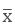of n values x1, x2, x3, ...... xn is given by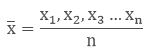Median

If we arrange the numbers in an ascending or descending order then the middle number of the series will be median. If the number of series is even then the median will be the average of two middle numbers.

If n is odd then the median isobservation.

If the n is even then the median is the average of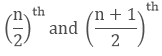observation.

Mode

The number which appears most frequently in the series then it is said to be the mode of n numbers.

Mean of Grouped Data (Without Class Interval)

If the data is organized in such a way that there is no class interval then we can calculate the mean by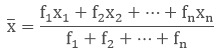where, x1, x2, x3,...... xn are the observations

f1, f2, f3, ...... fn are the respective frequencies of the given observations.

Example

Grouped Population Mean

x
f
fx

20
40
800

40
60
2400

60
30
1800

80
50
4000

100
20
2000

200
∑fx = 11000

Here, x1, x2, x3, x4, x5 are 20, 40, 60, 80, 100 respectively and f1, f2, f3 , f4, f5 are 40, 60, 30, 50, 20 respectively.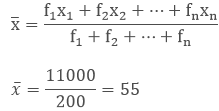Mean of Grouped Data (With Class-Interval)

When the data is grouped in the form of class interval then the mean can be calculated by three methods.

1. Direct Method

In this method, we use a midpoint which represents the whole class. It is called the class mark. It is the average of the upper limit and the lower limit.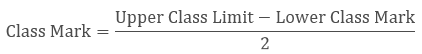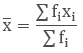Example

A teacher marks the test result of the class of 55 students for mathematics. Find the mean for the given group.

Marks of Students
0 – 10
10 – 20
20 – 30
30 – 40
40 – 50
50 – 60

Frequency
27
10
7
5
4
2

To find the mean we need to find the mid-point or class mark for each class interval which will be the x and then by multiplying frequency and midpoint we get fx.

Marks of students
Frequency(f)
Midpoint(x)
fx

0 – 10
27
5
135

10 – 20
10
15
150

20 – 30
7
25
175

30 – 40
5
35
175

40 – 50
4
45
180

50 – 60
2
55
110

∑f = 55
∑fx = 925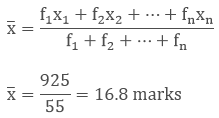2. Deviation or Assumed Mean Method

If we have to calculate the large numbers then we can use this method to make our calculations easy. In this method, we choose one of the x’s as assumed mean and let it as “a”. Then we find the deviation which is the difference of assumed mean and each of the x. The rest of the method is the same as the direct method.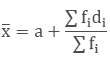Example

If we have the table of the expenditure of the company's workers in the household, then what will be the mean of their expenses?

Expense(Rs.)
100 - 150
150 - 200
200 - 250
250 - 300
300 - 350
350 - 400

Frequency
24
40
33
28
30
22

Solution

As we can see that there are big values of x to calculate so we will use the assumed mean method.

Here we take 275 as the assumed mean.

Expenses(Rs.)
Frequency(f)
Mid value(x)
d = x – 275
fd

100 – 150
24
125
- 150
- 3600

150 – 200
40
175
- 100
- 4000

200 – 250
36
225
- 50
-1650

250 – 300
28
275
0
0

300 – 350
30
325
50
1500

350 – 400
22
375
100
2200

∑f = 180

∑fd = - 5550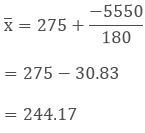3. Step Deviation Method

In this method, we divide the values of d with a number "h" to make our calculations easier.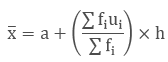Example

The wages of the workers are given in the table. Find the mean by step deviation method.

Wages
20 - 30
20 - 30
30 - 40
40 - 50
50 - 60

No. of workers
8
9
12
11
6

Solution

Wages
No. of workers (f)
Mid-point(x)
Assume mean (a) = 35, d = x - a
h = 10, u = (x – a)/h
fu

10 – 20
8
15
-20
-2
-16

20 – 30
9
25
-10
-1
-9

30 – 40
12
35
0
0
0

40 – 50
11
45
10
1
11

50 – 60
6
55
20
2
12

∑f = 46

∑fu = -2Mode of Grouped Data

In the ungrouped data the most frequently occurring no. is the mode of the sequence, but in the grouped data we can find the class interval only which has the maximum frequency number i.e. the modal class.

The value of mode in that modal class is calculated byl = lower class limit of the modal class

h = class interval size

f1 =frequency of the modal class

f0 =frequency of the preceding class

f2 = frequency of the succeeding class

Example

The table of the marks of the students of a class is given. Find the modal class and the mode.

Marks
0 – 20
20 – 40
40 – 60
60 – 80
80 – 100

No. of students
4
8
6
7
5

Solution

Here we can see that the class interval with the highest frequency 8 is 20 – 40.

So this is our modal class.

Modal class = 20 - 40

Lower limit of modal class (l) = 20

Class interval size (h) = 20

Frequency of the modal class(f1) = 8

Frequency of the preceding class(f0) = 4

Frequency of the succeeding class (f2) = 6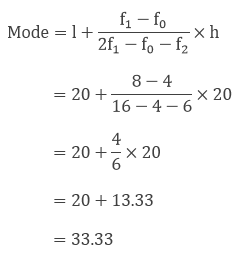Median of Grouped Data

To find the median of a grouped data, we need to find the cumulative frequency and n/2

Then we have to find the median class, which is the class of the cumulative frequency near or greater than the value of n/2.

Cumulative Frequency is calculated by adding the frequencies of all the classes preceding the given class.

Then substitute the values in the formulawhere l = lower limit of median class

n = no. of observations

cf = cumulative frequency of the class preceding to the median class

f = frequency of the median class

h = size of class

Example

Find the median of the given table.

Class Interval
Frequency
Cumulative Frequency (fc)

1 – 5
4
4
4

6 – 10
3
7
4 + 3 = 7

11 – 15
6
13
7 + 6 = 13

16 – 20
5
18
13 + 5 = 18

21 – 25
2
20
18 + 2 = 20

N = 20

Solution

Let’s find the n/2.

n = 20, so n/2 = 20/2 = 10

The median class is 11 - 15 as its cumulative frequency is 13 which is greater than 10.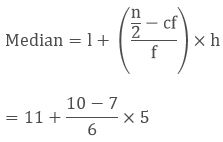13.5

Remark: The empirical relation between the three measures of central tendency is

3 Median = Mode + 2 Mean

Graphical Representation of Cumulative Frequency Distribution

The graph makes the data easy to understand. So to make the graph of the cumulative frequency distribution, we need to find the cumulative frequency of the given table. Then we can plot the points on the graph.

The cumulative frequency distribution can be of two types -

1. Less than ogive

To draw the graph of less than ogive we take the lower limits of the class interval and mark the respective less than frequency. Then join the dots by a smooth curve.

2. More than ogive

To draw the graph of more than ogive we take the upper limits of the class interval on the x-axis and mark the respective more than frequency. Then join the dots.

Example

Draw the cumulative frequency distribution curve for the following table.

Marks of students
0 – 10
10 – 20
20 – 30
30 – 40
40 – 50
50 – 60

No. of students
7
10
14
20
6
3

Solution

To draw the less than and more than ogive, we need to find the less than cumulative frequency and more than cumulative frequency.

Marks
No. of students
Less than cumulative frequency
More than cumulative frequency

0 – 10
7
Less than 10
7
More than 0
60

10 – 20
10
Less than 20
17
More than 10
53

20 – 30
14
Less than 30
31
More than 20
43

30 – 40
20
Less than 40
51
More than 30
29

40 – 50
6
Less than 50
57
More than 40
9

50 – 60
3
Less than 60
60
More than 50
3

More than 60
0

Now we plot all the points on the graph and we get two curves.Remark

The class interval should be continuous to make the ogive curve.

The x-coordinate at the intersection of the less than and more than ogive is the median of the given data.

```### Course Features

• 728 Video Lectures
• Revision Notes
• Previous Year Papers
• Mind Map
• Study Planner
• NCERT Solutions
• Discussion Forum
• Test paper with Video Solution